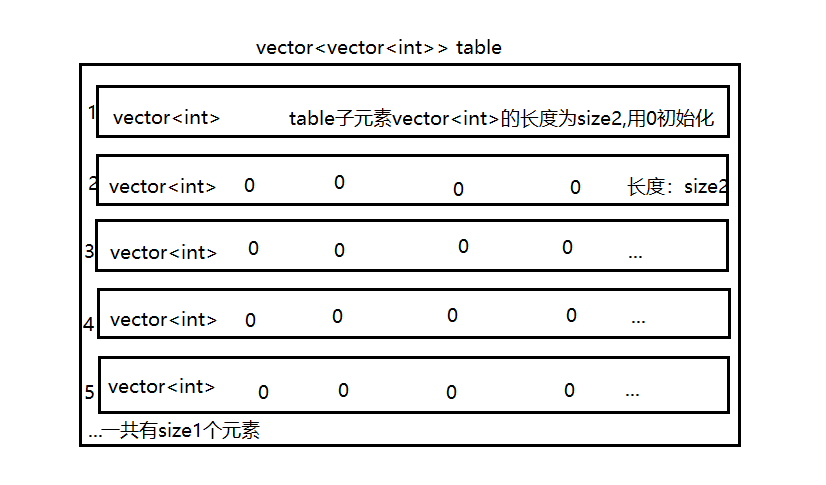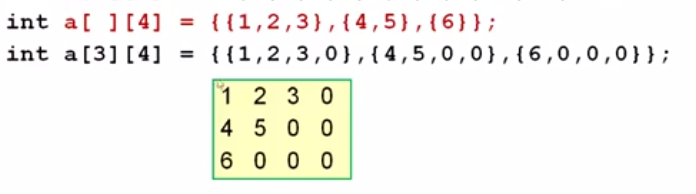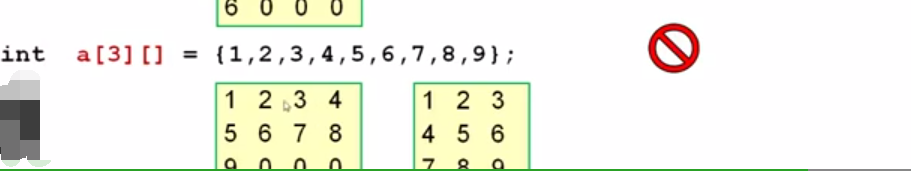• //一次for循环初始化三维数组 void main() { int num; for (int i = 0; i ; i++) { num[i / 12][i / 3 % 4][i % 3] = i + 1; } for (int i = 0; i ; i++) { for (int j = 0; j ; j++) { ...
#include<stdio.h>
#include<stdlib.h>

//一次for循环初始化三维数组
void main()
{
int num;

for (int i = 0; i < 60; i++)
{
num[i / 12][i / 3 % 4][i % 3] = i + 1;
}

for (int i = 0; i < 5; i++)
{
for (int j = 0; j < 4; j++)
{
for (int k = 0; k < 3; k++)
printf("%-3d", num[i][j][k]);
putchar('\n');
}
putchar('\n');
}

system("pause");
}
展开全文c语言 数组
• table，但是不知道怎么对其进行初始化初始化时指定二容器的大小）,于是网上搜索一番，最终找到了如下一行代码： vector<vector<int>> table(size1, vector<int>(size2, 0)); 代码说明：声明...
C++二维容器vector<vector>初始化方法解析
遇到的问题：
在解决“求最大字串”问题时想到了用二位数组vector<vector<int>> table，但是不知道怎么对其进行初始化（初始化时指定二维容器的大小）,于是网上搜索一番，最终找到了如下一行代码：
vector<vector<int>> table(size1, vector<int>(size2, 0));

代码说明：声明一个名为table的容器，其元素为vector的容器。简单来说类似一个int型的二维数组。
这样，就得到了一个如下图所示的二维容器。具体代码的内容，可以这样理解：图中，我将外围容器table的初始化参数分成了两部分A、B。
A: table外围容器的大小
B: table外围容器的内容，即size1个vector型的元素。
B1：内部容器的大小
B2：内部容器的内容
观察规律，可以得出如下的初始化格式：
容器（大小，内容）。
推广：三维容器
将数组的二维容器的初始化推广到三维容器。
定义一个长宽高为2x3x5的立方体容器，每个元素为0，我们得到如下代码，结果编译通过。
//长宽高：2*3*5
vector<vector<vector<int>>> cube(5, vector<vector<int>>(3, vector<int>(2, 0)));

画的很简陋，随便欣赏一下。补充内容：
1.声明一个简单int容器
vector<int> v;

2.声明一个长度为5的容器
vector<int> v(5);

3.声明一个长度为5，所有元素初始值为0的容器
vector<int> v(5,0);

4.用已有的数组初始化容器,区间：[a,a+6)
int a={5,6,2,0,9,4};
vector<int >  v(a,a+6);

5.用现有容器初始化一个容器
vector<int> v1(5,0);
vector<int> v2(v1);

6.用迭代器初始化容器
vector<int> v1(5, 0);
vector<int> v2(v1.begin(),v1.end());

杂谈：

在程序中，当再次向vector插入元素时，由于vector的容量不够，所以引起了内存的重新分配。
一旦内存重新配置，与之相关的所有的reference、pointers、iterators都会失效。原因：内存地址变动.


展开全文• 维数组初始化 （1）二维数组的初始化 int a[ ]={1,2,3,4,5,6,7,8,9,10,11,12} ; int a={1,2,3,4,5,6,7,8,9,10,11,12} ; int a={ {1,2,3},{4,5,6},{7,8,9},{10,11,12} } ; 这者赋值方式等价； 这...
二维数组初始化
（1）二维数组的初始化
int a[ ]={1,2,3,4,5,6,7,8,9,10,11,12} ;
int a={1,2,3,4,5,6,7,8,9,10,11,12} ;
int a={ {1,2,3},{4,5,6},{7,8,9},{10,11,12} } ;
这三者赋值方式等价；这个赋值方法是正确的；这种赋值方法是错误的，在java中会报java.lang.NullPointerException。对于这个定义而言，无法确定是3行几列，（c语言中二维数组是按行存放的，因此必须知道行数，才知道他的逻辑存储结构）


展开全文• //错误，如果提供了数组初始化操作，则不能定义表达式 int[] a; a = new int; //正确，与（2）相同 int[] a; a = {1,2,3,4,5}; //错误，数据常量只能在初始化操作中使用，如（3） int a[]; a = 1; a = 2...
文章目录一维数组与二维数组的声明一维数组的声明：二维数组的声明：总结数组的初始化静态初始化动态初始化默认初始化
一维数组与二维数组的声明
一维数组的声明：

int[] a;  //只声明数组a，没有初始化

int[] a = new int;  //初始化为默认值，int型为0

int[] a = {1,2,3,4,5};  //初始化为给定值

int[] a = new int[] {1,2,3,4,5};  //初始化为给定值
int[] a = new int {1,2,3,4,5};  //错误，如果提供了数组初始化操作，则不能定义维表达式

int[] a;  a = new int;  //正确，与（2）相同
int[] a;  a = {1,2,3,4,5};  //错误，数据常量只能在初始化操作中使用，如（3）

int a[];  a = 1;  a = 2;  //错误，因为数组没有初始化，不能直接赋值

二维数组的声明：

int[][] a;  //只声明数组a，没有初始化

int[][] a = new int;  //初始化为默认值，int型为0

int[][] a = {{1,2},{2,3},{3,4}};  //初始化为给定值
int[][] a = {{1,2},{2,3},{3,4,5}};  //正确，数组空间不是连续分配的，所以不要求每一维的空间大小相同

int[][] a = new int[];
a = new int;  // a其实就是一个一维数组
a = new int;  //每一维的大小可以不一样

int[][] a = new int[][]{{1,2},{2,3},{3,4,5}};  //正确，与（3）相同
int[] a = new int{{1,2},{2,3},{3,4,5}};  //错误，如果提供了数据初始化操作，则不能定义维度表达式
int[][] a = new int[];  a = {1,2,3,4,5};  //错误，数组常量只能在初始化操作中使用

int[][] a = new int[];  a = 1;   //错误，第二维没有初始化，不能赋值。出现java.lang.NullPointerException异常

总结

数组是对象类型数据，声明数组时注意new的使用 二维数组就是数组的数组，里面的数组大小不要求一样
不管是一维数组还是二维数组，在使用前（赋值，访问）必须初始化，可以使用new默认初始化，也可以用数组常量初始化
声明数组是，一定要考虑数组的最大容量，防止容量不够的现象。数组一旦被声明，它的容量就固定了，不容改变。如果想在运行程序时改变容量，就需要用到数组列表
数组有一个缺点，就是一旦声明，就不能改变容量，这个也是其使用率不高的原因，一般存储数据会使用数组列表或vector，这两种数据结构来存储数据

数组的初始化

动态初始化：数组定义与为数组分配空间和赋值操作分开进行(静态初始化是在声明数组的同时进行辅助，动态初始化是在先声明数组，然后再对其进行赋值)
静态初始化：在定义数组的同时就为数组元素分配空间并赋值(静态初始化是在声明数组的同时进行辅助，动态初始化是在先声明数组，然后再对其进行赋值)
默认初始化：数组是引用类型，它的元素相当于类的成员变量，因此数组分配空间后，每个元素也被按照成员变量的规则被默认初始化

静态初始化
int intArray[] = {1,2,3,4};
String stringArray[] = {"abc" ,"bcd", "cde" };

//TestS.java（静态）
public class TestS{
public static void main(String[] args) {
int[] a = {1,2,3};
Time times[] = {new Time(15,53,23),new Time(15,52,24),new Time(15,51,25)};
System.out.println("a = " + a + "\n" + "a = " + a + "\n"  + "a = " + a);
}
}

class Time{
int hour,min,sec;
Time(int hour,int min,int sec){
this.hour = hour;
this.min = min;
this.sec = sec;
}
}

动态初始化
int intArray[];    intArray = new int;
String stringArray[];    stringArray = new String;  //为数组中每个元素开辟引用空间（32位）
stringArray = new String("How");  //为第一个数组元素开辟引用空间（32位）
stringArray = new String("are");  //为第二个数组元素开辟引用空间（32位）
stringArray = new String("you");  //为第三个数组元素开辟引用空间（32位）

//TestD.java（动态）
public class TestD{
public static void main(String[] args) {
int[] a;
a = new int;
a = 0;
a = 1;
a = 2;

Date days[];
days = new Date;
days = new Date(2019,1,7);
days = new Date(2019,1,8);
days = new Date(2019,1,9);

System.out.println("days = "+days + "\n" +"days = "+days + "\n"+"days = "+days);
}
}
class Date{
int year,month,day;
Date(int year,int month,int day){
this.year = year;
this.month = month;
this.day = day;
}
}

默认初始化
//TestDefault（默认）
public class TestDefault{
public static void main(String[] args) {
int[] a = new int;
System.out.println(""+a);
}
}



展开全文• 下面主要介绍下二位数组初始化种方式 1、定义数组的同时使用大括号直接赋值，适合数组元素已知的情况 2、定义二维数组的大小，然后分别赋值 3、数组第二维的长度可变化 //第一种方式：定义的同时大括号...java
• 这里介绍C#二维数组初始化，C#数组并不是动态的，你必须自己来把他变成动态结构，除非你使用其他类。C#二维数组初始化，有两种方法。 在向大家详细介绍C#二维数组初始化之前，首先让大家了解下C#多维数组，然后全面...c# matrix system class
• package libai; public class meihua { public static void main(String[] args) { // TODO Auto-generated method stub char a[][]=new char[]; //数组初始化 a=new char[]{'云','想','衣'...
• 维数组初始化和找最大值二维数组初始化二维数组找最大值 二维数组初始化 我们先看一维数组初始化 这是一个一维数组, 里面的每一个元素，都是一个基本类型 int int a[] =new int[]{1,2,3,4,5}; 接下来看二维数组...java
• （二）二维数组初始化格式 1.动态初始化 1.1 二维数组格式1 数据类型[][] 变量名 = new 数据类型[m][n]; m表示这个二维数组有多少个一维数组 必须写上 n表示每一个一维数组的元素个数 可选 举例: int[][] arr = new ...java
• 1）静态数组 int array2={{4,2},{5},{6}};...以上初始化完成，后续赋值及使用 例： array2=1 ； int t=array2; 释放二维数组 for (int i = 0; i < m;i++) { delete[]a
• 数据类型 数组名[整常量表达式][ 整常量表达式]={ 初始化数据 }； 在{ }中给出各数组元素的初值，各初值之间用逗号分开。把{ }中的初值依次赋给各数组元素。 有如下几种初始化方式： ⑴ 分行进行初始化 int a=...C
• ![图片说明](https://img-ask.csdn.net/upload/201711/06/1509937541_702321.png)  #include #include int main() { int i; char s={"12345","12345"}; for(i=0;i;i++) { printf("%s\n",s[i]);...c
• #region 二维数组 //https://www.cnblogs.com/zblc2016/p/5721725.html using System; using System.Collections; using System.Collections.Generic; using System.Drawing; using System.IO; using System.Linq; ...
• 1.定义数组直接赋值 int a[]= {1,2,3,4,5}; 2.用new在数组中创建元素 int a[]=new int[]{1,2,3}; 注意：后面的[]不要忘...输出结果为[0, 0, 0]，Arrays.toString产生一维数组的可打印版本。 4.先声明再赋值 int cjava 编程语言
• /* 第一种方式 */ int tdarr1[][] = { { 1, 3, 5 }, { 5, 9, 10 } }; /* 第二种方式 */ int tdarr2[][] = new int[][] { { 65... /* 第种方式 */ int tdarr3[][] = new int; // 先给数组分配内存空间 ...
• C语言的二维数组初始化的几种方式介绍1、直接赋值2、循环对每个元素赋值3、借用memset/memset_s初始化为0或-14、数组所有元素初始化为相同值` 1、直接赋值 适合数组元素较少的，各元素的值可以不同。 int arr...数据结构
• float a; float b; Mat A = Mat (2*g_maxCornerNumber,11, CV_64F,a); Mat B = Mat (2*g_maxCornerNumber,1, CV_64F,b);... 这是初始化的代码，可是输出的全是乱码，是怎么回事，求大神指点！opencv 特征点
• #include #include int main() { int **arr; int m=3,n=4,i,j; arr = (int **)malloc(sizeof(int*)*m); for(i=0; i; i++) arr[i] = (int *)malloc(sizeof(int)*n);c语言 malloc sizeof
• * 问题：定义一个三维数组，并初始化之。写一个打印函数，负责打印三维数组中的数据，并测试。 */ public class Test11 { public static void main(String[] args) { int[][][] arr = new int[][]; //对第一...
• ## 三维数组的初始化

千次阅读 2015-03-17 15:26:01
int[,,]=new int[2,3,4]{ {{1,2,3,4}{1,2,3,4}{1,2,3,4}} {{5,6,7,8}{5,6,7,8}{5,6,7,8}} } 注意初始化要先从最外围开始。c语言
• ## 二维数组正确初始化规则

千次阅读 多人点赞 2019-03-19 20:30:50
最近刷题总在二维数组初始化这里栽跟头，接下来总结一点二维数组的初始化规则以便记忆 这里提一句一维数字代表行，二维数字代表列 arr就是创建两行列的数组 二维数组在初始化的时候可以分行进行初始化 int...
• /* *三维数组初始化及遍历 * */ public class test { public static void main(String[] args) { int a[][][]=new int[][][]{ {{1,2,3},{4,5,6}}, {{8,9,10},{11,12,13}}, {{14,15,16...
• 用变量初始化三维数组初始化二维原理一样，只是在二维的基础上每一个元素纵向扩充。 #include "stdafx.h" #include using namespace std; int _tmain(int argc, _TCHAR* argv[]) { int a = 3, b = 3, ...
• 1. 向一个函数的参数传递二维数组种方法： #include // // 第一种传递方式（指针方式）：int (*x) // 内部可以把x当成一个二维数组使用 // void Fn1(int (*x)) { for (int i=0; i; i++) { for...
• ## 数组初始化

千次阅读 2018-07-05 16:02:49
一：一维数组初始化1、a = {0}; // 可以看做第一个元素初始化为0，其余元素没有初始化，默认为0，最后所有元素为02、a = {1, 2, 3}; //a=1,a=2,a=3,其余为03、a = {1, 2, 3,}; //这里单独拿出来，...c
• 指针数组初始化 行数为：row 列数为：column 方式一： int **dp1; dp1 = (int **)malloc(sizeof(int *) * row);//分配指针数组，计算行的大小 for(int i=0; i&lt;row; i++) { dp1[i] = (int *)malloc...C语言...